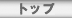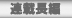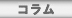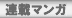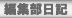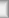# \$BEZ\$r7!\$k\$HN_!9\$H%;%-%;%\$%\$%s%3\$N;`3<\$,\$G\$F\$/\$k(J

A corpse of a lot of parakeets appeared when I dug soil.

## doru

\$B!!2f\$,2H\$NDm\$O%Z%C%H!&%;%a%?%j!<2=\$7\$F\$\$\$^\$9!#%;%-%;%\$%\$%s%3\$NB>\$K6e41D;\$H\$+7\\$N9|\$b\$G\$F\$-\$^\$9!#(J
\$B\$_\$s\$J7lFy\$OIe\$j!"\$_\$s\$J
\$B7G:\9f(J
\$B%?%\$%H%k(J
HTML
\$BBh#1#2#99f(J
\$BEZ\$r7!\$k\$HN_!9\$H%;%-%;%\$%\$%s%3\$N;`3<\$,\$G\$F\$/\$k(J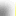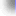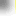\$B%H%C%W(J\$B!?(J\$BFI@ZC;JT(J\$B!?(J\$BO":\D9JT(J\$B!?(J\$B%3%i%`(J
\$B%V%C%/%l%S%e!<(J\$B!?(J\$BCx\$B!?(J\$BO":\%^%s%,(J\$B!?(JBBS\$B!?(J\$BJT=8ItF|5-(J
\$BCx:n8"(J\$B!?(J\$B%W%i%\$%P%7!<%]%j%7!<(J\$B!?(J\$B%5%\$%H%^%C%W(J